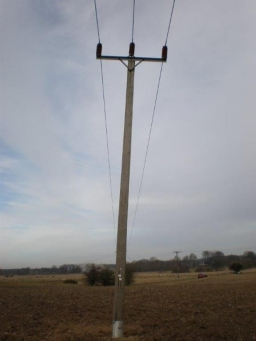# Length 40391

The wheels are stuck in the water. 40 cm is in the ground, 2/3 of the length is in the water, and 1/5 of the length is above the water. How long is the wheel, how many cm is in the water, and how much is above the water?

x =  300 cm
a =  200 cm
b =  60 cm

### Step-by-step explanation:Did you find an error or inaccuracy? Feel free to write us. Thank you!

Tips for related online calculators
Need help calculating sum, simplifying, or multiplying fractions? Try our fraction calculator.
Do you have a linear equation or system of equations and looking for its solution? Or do you have a quadratic equation?
Do you want to convert length units?